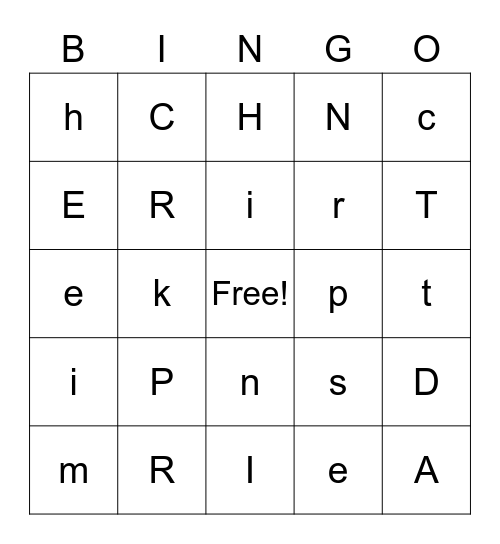# K17This bingo card has a free space and 52 words: S, A, T, I, P, N, C, K, E, H, R, M, D, s, a, t, i, p, n, c, k, e, h, r, m, d, S, A, T, I, P, N, C, K, E, H, R, M, D, s, a, t, i, p, n, c, k, e, h, r, m and d.

⚠ This card has duplicate items: S (2), A (2), T (2), I (2), P (2), N (2), C (2), K (2), E (2), H (2), R (2), M (2), D (2), s (2), a (2), t (2), i (2), p (2), n (2), c (2), k (2), e (2), h (2), r (2), m (2), d (2)

## Play Online